# Effects of tangential and radial velocity on the heat transfer for flow through pipe with twisted tape insert-turbulent flow

Effects of tangential and radial velocity on the heat transfer for flow through pipe with twisted tape insert-turbulent flow

Parag Chaware C.M. Sewatkar

Department of Mechanical Engineering, College of Engineering Pune 411005, India

Department of Mechanical Engineering, MKSSS's Cummins college of Engineering for Women, Pune 411052, India

Department of Mechanical Engineering, College of Engineering Pune 411005, India

Corresponding Author Email:
cms.mech@coep.ac.in
Page:
811-820
|
DOI:
https://doi.org/10.18280/ijht.350417
|
Accepted:
|
Published:
31 December 2017
| Citation

OPEN ACCESS

Abstract:

Numerical analysis of heat transfer improvement in a pipe with the twisted tape in the turbulent regime is carried out for twist ratios of 4, 5, 6, 8 and 10 at 4000 ≤ Re ≥ 30000. The velocity field in terms of axial, tangential and radial velocity and temperature field are studied with the twist severity and Reynolds number as governing parameters.  The heat transfer augmentation with insertion of twisted tape predominantly comes from the tangential and radial velocity. The tangential velocity significantly alters the flow field and hence the heat transfer process. The tangential velocity increases with the distance from the center of the pipe and affects the thermal and velocity boundary layer. The radial velocity is responsible for radial convection. The numerical results are correlated for the friction factor and Nusselt number. The correlations show good resemblance with the experimental data.

Keywords:

heat transfer augmentation, radial velocity, tangential velocity, twisted tape

1. Introduction

Swirl flow promoting devices are used for enhancing the heat transfer across many industries. It finds the applications in process industry, chemical industry and casting processes to homogenize the mixture. The swirl-flow devices are passive technique. It consists of a variety of tube inserts include twisted tapes, helical tapes, periodically spaced propellers. Twisted tape is a metallic strip twisted along their longitudinal axis at desired dimensions.  The low cost and ease of retrofitting to the existing system makes it the most favorable tube insert technique for generating swirl flow.

Twisted tapes are widely investigated by many researchers since the twentieth century. Smithberg and Landis  are the first ever to predict flow profile, of turbulent. Their analysis led them to develop predictive correlations for pressure drop and heat transfer as friction factor and Nusselt number. They characterized the full length twisted tape generated swirl flow as core flow helicoidal in nature with secondary circulation. The boundary layer flow appears to be twisting in order of the twist. They found that, except for the near wall region where the boundary layer effects are predominant, the axial velocity remained almost constant over the cross section.  The performance analysis of the twisted tape is investigated both experimentally and numerically by Hong and Bergles  in the laminar regime. They argued that the increase in secondary velocities and modified structure of thermal boundary layer are reasons for the heat transfer enhancement. Date  reported that the tape fin effect arising from conductivity of the tape material is the key parameter in heat transfer enhancement.

Correlations based on empirical study were proposed by Manglik and Bergles for laminar flow  and turbulent flow  using Reynolds number and twisted ratio as the governing parameters.  Their study also shows the monotonic transition, prohibiting the transition jump. transition.  A new nondimensional parameter 'Swirl Number' to define the flow regimes for swirling flow generated by twisted tape is also proposed by them.

Effect of tube tape clearance was studied by Sami AI-Fahed and Walid Chakroun  and Halit Bas and Veysel Ozceyhan . Their study shows that tube tape clearance has lesser contribution in heat transfer than the severity of the twist and the width of the tape should in perfect fitness with the tube for achievement of the maximum heat transfer efficiency. Performance comparison of the twisted tape with other turbulent promoter like wire coil insert is carried out by Wang and Sunden  in the turbulent regime. They conclude that, twisted tape is a better heat transfer enhancement option over wire coil inserts of the equal thickness ratio and helix angle.

To achieve the reduction in high pressure, drop associated with the twisted tape insert spread over the pipe length, the other forms like small length twisted tapes and evenly spaced small length twisted tapes are also investigated. Klepper  used the shorter length twisted tape instead of full length and inferred that the pressure drop penalty can be reduced with the use of such arrangement. However, decaying swirl over the length reduces enhancement advantage.  Saha et al.   studied the arrangement of multiple short length twisted tape. The performance of evenly spaced twisted tapes, which provides periodical swirl, mainly depends on the space ratio and the phase angle between two adjacent twisted tape elements. This arrangement can provide less pressure drop than full length twisted tapes. The better heat transfer performance with short length tapes is found in laminar regime over the turbulent. Eiamsa-ard et al.  also studied the evenly spaced twisted tape and showed that the free space ratio of lesser than one has to be achieved for greater heat transfer rate.  Cazan and Aidun  performed the experimental analysis of the swirling flow downstream the short twisted tapes. According to the experimental measurements, they proposed the vortex generation mechanism inside the tube with twisted tape. According to their proposition, two vortices of secondary flow are induced, a weaker counter-rotating vortex with the strong corotating vortex. The tangential velocity accelerates the corotating vortex. It expands while the other counter-rotating vortex diminishes. The LDV measurement carried out by them also does not show any evidence of weaker counter rotating vortex. Bhattacharyya   et al.  reported the investigations of centre trimmed twisted tape. In such cases the heat transfer augmenttation is more significant than pressure drop penalty associated with it.

Numerical study of rib as turbulent promoters in square duct is presented by Shukla and Dewan . The k-ε turbulence model and its two other variations namely RNG and Realizable are used for the performance comparison. All the three models are found suitable to predict the recirculation zone after the rib. Numerical study of twisted tape performance was carried out by Date and Saha . They observed that the axial velocity profile becomes flatter with smaller twist ratio and rise in Reynolds number. The modified forms of the twisted tapes shows better performance over the conventional tapes in terms of heat transfer. Chiu et al.  compared the implementation of twisted tape with two modified forms, longitudinal tape, and longitudinal tape with holes.  Their investigations showed that the longitudinal tape offers good results same as the classic tape with the maximum area reduction at the lowest twist ratio.  Rahimi et al.  presented the comparison of three types of modified forms. The jagged' type of twisted tape shows maximum heat transfer and performance ratio. The turbulence intensity produced by the jagged tape and the vorticity at the jagged edges is also the key factor in producing the highest heat transfer among the type of tapes studied. Kaliakatsos et al.  has compared the relative effectiveness of twisted tape with the oil and water as working fluid.

It can observe from the literature survey that, most of the studies carried out by earlier investigators report the experimental investigations. Their primary focus is to predict the effect of flow velocity and severity of twist on the heat transfer enhancement. The computational studies on short length tapes and modified twisted tapes are also reported in the literature. The computational studies using short length twisted tapes are primarily done for the optimization of the space ratio. The numerical analysis of the modified forms of the twisted tape report the performance enhancement factors and heat transfer characteristics. Thus, it is noted that the phenomenon of heat transfer enhancement with the employment of twisted tape is widely studied.  However, due to inherent limitations of experimental measurements and complexity of flow the detailed physical mechanism of heat transfer process for the turbulent regime is not found in the literature. The factors responsible for high heat transfer like swirl, tangential, radial and axial velocity distribution, the temperature distribution can be analyzed with the help of numerical simulations. This may helpful in explaining the physics of heat transfer mechanism and the design of much more effective tape inserts. Thus, the important aim of this investigation is the qualitative and qualitative examination of variation of axial, radial and tangential velocities over a particular section with Reynolds number and twist ratio. The significance of these parameters on heat transfer augmentation is also studied.

2. Description of the Physical Domain

The performance of the twisted tape is analyzed using the test section arrangement shown in Figure 1. The twisted tape of negligible thickness is inserted over the full length of the tube of diameter (D). The tape has a pitch H'. The pitch is defined for the 180° tape rotation. To ensure the perfect fitness of the tape with tube, the width `W' of the tape is same as the diameter of the tube. The perfect contact between tube and tape is maintained over the entire length. The orientation of the tape is repeated in the axial direction at ‘2H’ distance with respect to the tube. Hence the flow is periodically fully developed in the axial direction in the tube  .

## 1.pngFigure 1. Twisted tape geometry

The grid used in the present analysis is shown in Figure 2. The domain is discretized into hexahedral cells with Water as a working fluid. The fluid compressibility and variation in properties is assumed to be negligible for the given flow conditions.  The flow and heat transfer are in the steady state with no viscous dissipation. The grid size influence on the numerical results is also studied to confirm the optimum mesh size for the solution.

3. Computational Procedure

3.1 Grid generation

The computational grid is generated using GAMBIT as shown in Figure 2.   A smooth circular pipe is considered for the analysis. A twisted tape of the equal width of different is inserted in the pipe over its entire length. Length to diameter ratio is considered as four times the twist ratio. Hybrid mesh is created on the inlet and linked with the outlet. The grid over the tape surface and pipe wall is made finer to capture the boundary effects and maintaining the y+ value close to unity. The dimensions of the geometry are summarized in the Table 1.

Table 1. Geometry dimensions

 Geometry Dimension Diameter of the pipe 0.025m Width of the twisted tape 0.025m Length of the pipe and twisted tape 4 X Twist ratio Twist ratio 4,5,6,8 and 10

3.2 Boundary conditions

The present investigations consider the flow to be periodic, incompressible and fully developed turbulent in three dimensions. The no slip boundary condition was imposed over the pipe wall as well as on the surface of the twisted tape.  Translational periodic boundary condition is imposed at the inlet and outlet with constant mass flow rate and temperature of 300K.

## 2.png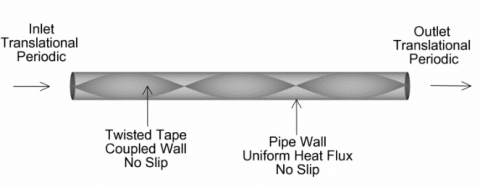Figure 2. Computational domain with boundary conditions

Constant surface heat flux (UHF) order of 2000 W/m2 is enforced on the tube wall with no slip condition. The tape is assumed as coupled wall. The thickness of the tube, tape and the contact resistance between tube and tape is neglected. Water is used as a working fluid and the properties of the water are assumed to be constant over the range of temperature studied. The flow and heat transfer are assumed to be steady without viscous dissipation. Investigations are carried out for twist ratio (H/D) 4, 5, 6, 8 and 10 in the turbulent region of flow regime at 4000 ≤ Re ≥ 30000. The grid independence study is carried out to confirm the optimum mesh size for the solution.

3.3 Solution procedure

In the present numerical solution, the incompressible and steady Navier–Stokes equations are employed. The continuity and Navier Stokes equations in Cartesian tensor notation can be written as;

$\frac{\partial}{\partial x_{i}}\left(\rho u_{i}\right)=0$             (1)

$\frac{\partial}{\partial x_{j}}\left(\rho u_{i} u_{j}\right)=-\frac{\partial p}{\partial x_{i}}+\frac{\partial}{\partial x_{j}}\left[\mu\left(\frac{\partial u_{i}}{\partial x_{j}}+\frac{\partial u_{j}}{\partial x_{i}}-\frac{2}{3} \delta_{i j} \frac{\partial u_{l}}{\partial x_{i}}\right)\right]$

$+\frac{\partial}{\partial x_{j}}\left(-\rho \overline{u_{i}^{\prime} u_{j}^{\prime}}\right)$                (2)

The term $-\rho \overline{u_{i}^{\prime} u_{j}^{\prime}}$  arising from fluctuating componenet of the velocity need to be modelled. The RSM model is capable of handling the anisotropic nature of the turbulence in the swirling flow. Kitoh's experimental and numerical study shows that the results obtained with RSM are in good agreement with the experimental results. To capture the wall effects, enhanced wall treatment with y+ ~1 is implemented. The Reynolds stress model calculates the individual Reynolds stresses which obviates the isotropy assumption of the Reynolds stresses. The Reynolds stresses in the above equation can be rewritten as;

\begin{align} & \frac{\partial }{\partial {{x}_{k}}}(\rho {{u}_{k}}\overline{{{{{u}'}}_{i}}{{{{u}'}}_{j}}})=-\ \ \frac{\partial }{\partial {{x}_{k}}}\left[ \rho \ \overline{{{{{u}'}}_{i}}{{{{u}'}}_{j}}{{{{u}'}}_{k}}}+\overline{p\left( {{\delta }_{kj}}{{{{u}'}}_{i}}+{{\delta }_{ik}}{{{{u}'}}_{j}} \right)} \right]+ \\ & \frac{\partial }{\partial {{x}_{k}}}\left[ \mu \frac{\partial }{\partial {{x}_{k}}}(\overline{{{{{u}'}}_{i}}{{{{u}'}}_{j}}}) \right]-\ \rho \left( \overline{{{{{u}'}}_{i}}{{{{u}'}}_{k}}}\ \frac{\partial {{u}_{j}}}{\partial {{x}_{k}}}+\ \overline{{{{{u}'}}_{j}}{{{{u}'}}_{k}}}\ \frac{\partial {{u}_{i}}}{\partial {{x}_{k}}} \right) \\ & +\overline{p'\left( \frac{\partial {{{{u}'}}_{i}}}{\partial {{x}_{j}}}+\frac{\partial {{{{u}'}}_{j}}}{\partial {{x}_{i}}} \right)}-2\mu \overline{\frac{\partial {{{{u}'}}_{i}}}{\partial {{x}_{k}}}\ \frac{\partial {{{{u}'}}_{j}}}{\partial {{x}_{k}}}} \\\end{align}             (3)

The equation (3) can be reprented as;

$C_{i j}=D_{T, i j}+D_{L, i j}+P_{i j}+\phi_{i j}-\varepsilon_{i j}$               (4)

where Cij is the Convective term, DT,ij equals the Turbulent Diffusion, DL,ij is molecular Diffusion,

Pij is the term for Stress Production, $\phi_{i j}$  is for the Pressure Strain, ɛij stands for the dissipation. Out of these DT,ij, $\phi_{i j}$ , and ɛij  are to be modeled for closing the equations. The above terms can be modeled as;

Turbulent Diffusion

$D_{T, i j}=\frac{\partial}{\partial x_{k}}\left(\frac{\mu_{t}}{\sigma_{k}} \frac{\partial \overline{u_{i}^{\prime} u_{j}^{\prime}}}{\partial x_{k}}\right)$               (5)

Pressure Strain

$\phi_{i j}=\phi_{i j, 1}+\phi_{i j, 2}+\phi_{i j, w}$             (6)

$\phi_{i j, 1} \equiv-C_{1} \rho \frac{\epsilon}{k}\left[\overline{u_{i}^{\prime} u_{j}^{\prime}}-\frac{2}{3} \delta_{i j} k\right]$               (7)

$\phi_{i j, 2} \equiv-C_{2}\left[\left(P_{i j}+F_{i j}+G_{i j}-C_{i j}\right)-\frac{2}{3} \delta_{j j}(P+G-C)\right]$

$P=\frac{1}{2} P_{k k}, G=\frac{1}{2} G_{k k}, C=\frac{1}{2} C_{k k}$                (8)

$\phi_{i j, w}=C_{1}^{\prime} \frac{\epsilon}{k}\left(\overline{u_{k}^{\prime} u_{m}^{\prime}} n_{k} n_{m} \delta_{i j}-\frac{3}{2} \overline{u_{i}^{\prime} u_{k}^{\prime}} n_{j} n_{k}-\frac{3}{2} \overline{u_{j}^{\prime} u_{k}^{\prime}} n_{i} n_{k}\right) \frac{C_{\ell} k^{3 / 2}}{\epsilon d}$

$+C_{2}^{\prime}\left(\phi_{k m, 2} n_{k} n_{m} \delta_{i j}-\frac{3}{2} \phi_{i k, 2} n_{j} n_{k}-\frac{3}{2} \phi_{j k, 2} n_{i} n_{k}\right) \frac{C_{\ell} k^{3 / 2}}{\epsilon d}$                  (9)

Dissipation

$\frac{\partial}{\partial t}(\rho \epsilon)+\frac{\partial}{\partial x_{i}}\left(\rho \epsilon u_{i}\right)=\frac{\partial}{\partial x_{j}}\left[\left(\mu+\frac{\mu_{t}}{\sigma_{\epsilon}}\right) \frac{\partial \epsilon}{\partial x_{j}}\right] C_{\epsilon 1} \frac{1}{2}\left[P_{i i}+C_{\epsilon 3} G_{i i}\right] \frac{\epsilon}{k}-C_{\epsilon 2} \rho \frac{\epsilon^{2}}{k}$                  (10)

Energy equation

$\frac{\partial}{\partial t}(\rho E)+\frac{\partial}{\partial x_{i}}\left[u_{i}(\rho E+p)\right]=\frac{\partial}{\partial x_{j}}\left[\left(k+\frac{c_{p} \mu_{t}}{\operatorname{Pr}_{t}}\right) \frac{\partial T}{\partial x_{j}}\right]$                        (11)

The turbulent heat transport in RSM is modeled using the concept of Reynolds' analogy to turbulent momentum transfer. The steady incompressible Navier–Stokes equations with the RSM model are solved by the finite volume method using solver FLUENT 17.1. Second order numerical schemes are applied for convective and diffusive term discretization. The convergence of the turbulence parameter is checked with the second order scheme. Since the flow is swirling in nature, the pressure field is evaluated using the PRESTO scheme. The pressure–velocity coupling SIMPLE (Semi Implicit Method for Pressure-Linked Equations) algorithm is used for pressure correction. The convergence is checked for the residual values below 10-6.  The Solutions are further iterated till the surface monitors of mean wall temperature, mean tangential velocity and bulk mean fluid temperature over a cross section in the in the axial direction shows the constant reading.  Data in the form of axial, tangential, radial velocities, pressure drop and temperature at various locations is obtained.

The data obtained numerically is validated with the empirical data by Manglik and Bergles  and Watanabe et al.  for pressure drop analysis and data by Manglik and Bergles  and  Sarma et al.  for heat transfer analysis. The comparison of the empirical and numerical friction factors is shown in Figure 3 and the comparison of empirical and numerical average Nusselt number are shown in Figure 4.  It is observed that numerical analysis results are in accord with the empirical data, with the maximum deviation about ±10%.

Correlations for the prediction of heat transfer enhancement and pressure drop and in terms of Nusselt number and friction factor based on numerical data are developed using non-linear regression analysis and are given by Eq. (7) to Eq. (11).

$f=22.48 \times f_{0} \times\left(\frac{H}{D}\right)^{-0.25}$ for $\quad \operatorname{Re}<10000$                 (7)

$f=20.4 \times f_{0} \times\left(\frac{H}{D}\right)^{-0.35}$ for $\quad R e>10000$                      (8)

where

$f_{0}=0.046 R e^{-02}$                (9)

For the Nusselt number results compared with the correlation, it is noted that the majority of numerical results show good agreement within 15%.

$N u=0.07 \times\left(1+\frac{1}{H / D}\right)^{1.25} \times R e^{0.7} \times P r^{0.4} for \quad R e<10000$                       (10)

$N u=0.06 \times\left(1+\frac{1}{H / D}\right)^{1.25} \times R e^{0.725} \times P r^{0.4} for \quad R e>10000$                     (11)

Resemblance of the data from present investigation and those calculated by the proposed correlations for Nusselt number Eq(5) and friction factor Eq(4) is shown in Figure 5. It can be observed that their good agreement, about 10%, between the numerical data and the data by proposed correlations.

## 3.png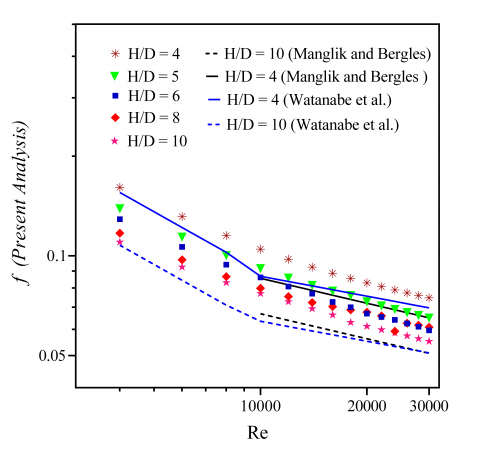Figure 3. Validation of the numerical results for friction factor

## 4.png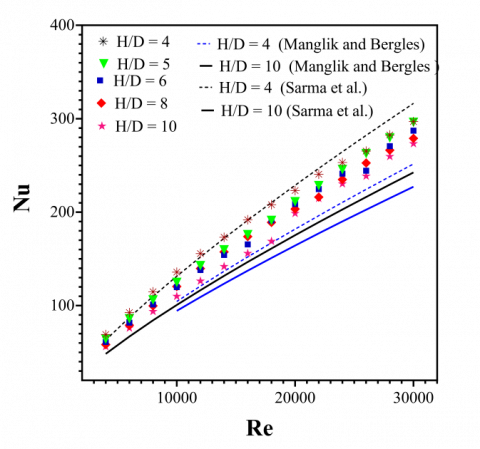Figure 4.Validation  of the numerical results for Nusselt number

## 51.png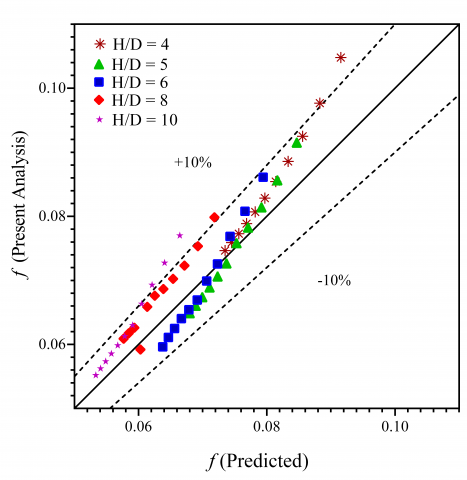(a)

## 52.png(b)

Figure 5. Resemblance of the (a) friction factor and (b) Nusselt number with the proposed correlations

4. Results

Insertion of the twisted tape alters the flow profile in a tube. The heat transfer augmentation using twisted tape is found to be a function of twist ratio and Reynolds number. Twisted tape imparts tangential and radial velocity components to the flow . The fluid temperature profile is also altered by the insertion of twisted tape .  The effects of Reynolds number and twist ratio on the distribution of axial, tangential and radial velocities, fluid temperature, pressure drop and heat transfer are studied and presented in this section. The heat transfer augmentation with the twisted tape depends on the severity of the twist defined by the twist ratio and Reynolds number. Twisted tape also imparts tangential and radial velocity components to the flow. It alters the flow and temperature profile of the fluid in a tube. The heat transfer augmentation is a result of the modified profile. This section discuss the influence of twist ratio and the Reynolds number on the distribution of axial, tangential and radial velocities, fluid temperature, pressure drop and heat transfer.

4.1 Axial velocity

Twisted tape converts the circular tube into two helical compartments. The flow takes place along the spiral path defined by the twist ratio in the tube. The axial velocity based Reynolds number is;

$R e=\frac{V_{z, m} D \rho}{\mu}$                   (12)

where, $V_{z, m}$ is the axial mean velocity.  Figures 6(a) to 6(d) show the contours of the axial velocity at Y = 4 and 10 for Re = 4000 and 30000, which are two extreme governing parameters.  The flow along the twisted tape can be divided as the helicoidal flow in the core and boundary layer flow. The magnitude of the maximum axial velocity decreases with increasing Reynolds number at a given twist ratio. This indicates the spread of core helicoidal flow region over the pipe cross section. For tighter twist the maximum velocity is observed at the tube tape contact area where the effect of inertia is predominant.  The least axial velocity is found in the vortex dominated area near the tape. However, shifting of the peak axial velocity towards the tape surface is found at tighter twist with increasing Reynolds number. This disturbs the thermal boundary layer over the tape surface and hence higher heat transfer from tape to the fluid or enhanced fin effect of the tape is achieved.

Figure 7 shows the axial velocity at an angle of π /2 to the tape surface, for different twist ratio at various values of Reynolds number.  The axial velocity profile is flatter at all Reynolds number for twist ratios 4,5 and 6. At the tighter twist the swirling nature of the flow, shifts the peak of axial velocity towards the tape. The shift of peak of velocity was also reported by Date . This in turn makes the velocity profile asymmetric. The asymmetric velocity profile further contributes the heat transfer augmentation by altering the boundary layer at the tube.

4.2 Tangential velocity

The tangential velocity contours are presented in Figure 8. The highest tangential velocity for tighter twist is a direct result of higher swirl imparted at tighter twist.  The core flow area near the tape is least affected by the swirl and hence the low tangential velocity is observed. At tighter twist the component of the tangential velocity is approximately 38% of the axial velocity. It decreases with increasing twist ratio. The highest tangential velocity component is only 17% for the twist ratio 10.

Thus, the severity of the twist is the governing parameter of the tangential velocity.  Intensity of secondary flow plays a key role in augmentation of heat transfer using twisted tape . The higher tangential velocity decreases the thermal gradients at the tube, increasing the heat transfer from tube to fluid. Therefore, higher heat transfer is found for the tighter twist ratios. With increasing twist ratio the swirling effect diminishes and hence the tangential velocity and heat transfer enhancement also reduces.

## 6.png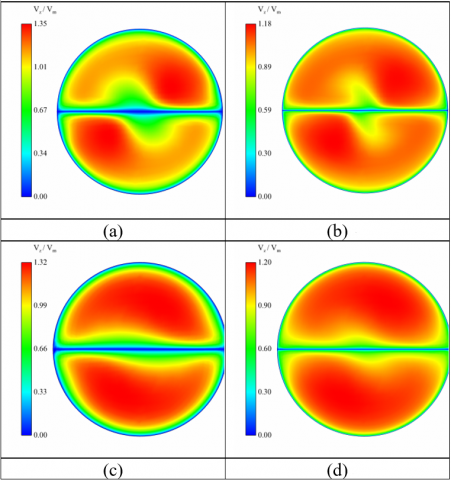Figure 6. Axial velocity contours for the fully developed flow at (a) Y = 4 and Re = 4000 (b) Y = 4 and Re = 30000 (c) Y = 10 and Re=4000 (d) Y = 10 and Re=30000.

## 71.png(a)

## 72.png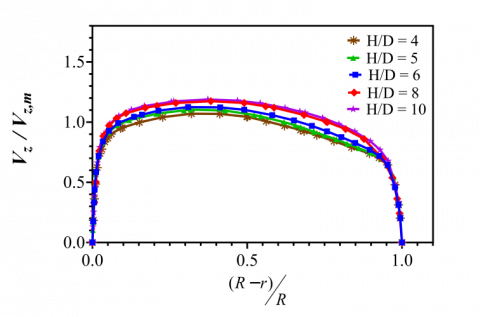(b)

Figure 7. Axial velocity profile plotted for fully developed flow at an angle of π/2 to the tape surface at (a) Re=4000 (b) Re=30000

Figure 9 shows the plots of tangential velocity plotted at an angle of π/2 to the tape surface at various Reynolds number. The tangential velocity increases with the distance from the tape towards the tube.  The highest tangential velocity is observed at the tube tape contact area in the direction of flow and near the tube. The rapid change in the direction in tube tape contact area increases the tangential velocity. The magnitude of tangential velocity for the given twist ratio is almost same for the range of Reynolds number studied. However, with increasing Reynolds numbers the point of highest tangential velocity shifts towards the tube surface. This shift is very similar to the axial velocity. The rise in radial component of the velocity shifts the peak towards the tube. The negative tangential velocity near the tape surface is the indicator of vortex formation near the tape.

## 8.png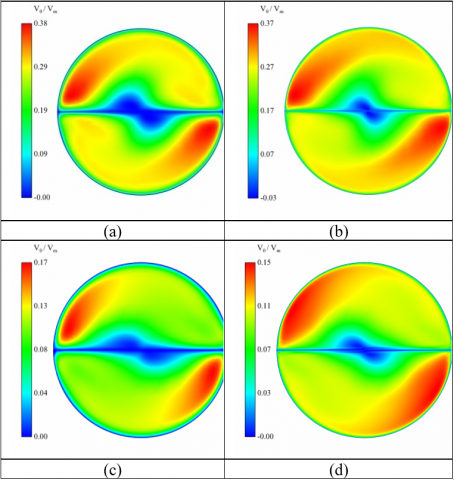Figure 8. Tangential velocity contours for the fully developed flow at (a) Y = 4 and Re = 4000 (b) Y = 4 and Re = 30000 (c) Y = 10 and Re=4000 (d) Y = 10 and Re=30000

## 91.png(a)

## 92.png(b)

Figure 9. Tangential velocity profile plotted at an angle of π/2 to the tape surface at (a) Re = 4000 (b) Re = 30000

Insertion of twisted tape also imparts radial velocity to the flow. The radial velocity is responsible for the cross flow mixing. Figure 9 shows the contours of the radial velocity. It is observed that the radial velocity component is lesser in magnitude than tangential velocity.  Higher radial velocity is observed at lower twists. This is the direct result of high rotational movement of the fluid particles at tighter twists and hence, more centrifugal action is experienced by the fluid particles.   The swirl in the flow decreases with increasing twist ratio and hence the radial and tangential velocity components also decrease. A continuous and rapid direction change occurs for the flow near the tape. Lesser centrifugal action, the dominance of boundary layer and rapid direction change decreases the radial velocity in the near tape area. The dominance of the radial velocity over the flow cross section decreases with increasing Reynolds number. The higher axial velocity reduces the radial component of the flow at the high Reynolds number.

Figure 10 shows the variation of radial velocity at an angle of π/2 to the tape surface at various Reynolds numbers. Similar to the tangential velocity maximum value of normalized radial velocity at the given twist ratio at all Reynolds number is almost same. However, unlike tangential velocity the peak of the radial velocity shifts towards the tape surface with increasing Reynolds number. The higher tangential velocity near the tube surface reduces the radial component. This shifts the radial velocity peak towards the tape surface. However, the presence of the boundary layer over the tape surface again decreases the radial velocity near the tape reducing the velocity gradients near the tape.

## 10.png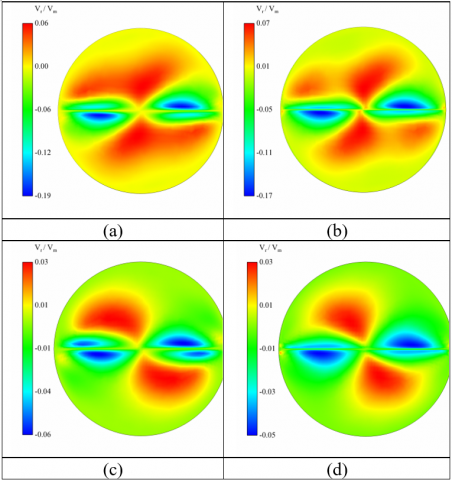Figure 10. Radial velocity contours for the fully developed flow at (a) Y = 4 and Re = 4000 (b) Y = 4 and Re = 30000 (c) Y = 10 and Re = 4000 (d) Y = 10 and Re=30000

## 111.png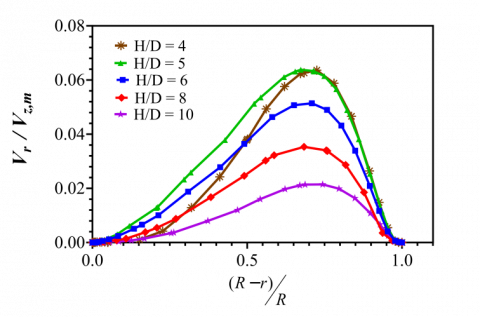(a)

## 112.png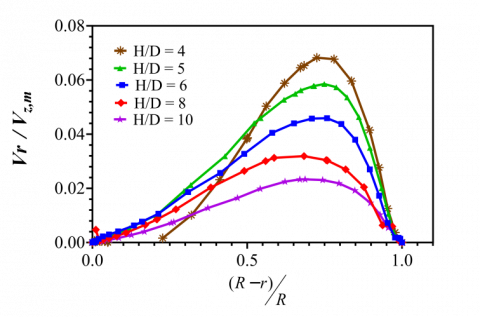(b)

Figure 11. Radial velocity profile plotted at an angle of π/2 to the tape surface at (a) Re = 4000 (b) Re = 30000

4.3 Temperature distribution

The twisted tape induced higher turbulence and swirl significantly alters the temperature profile. Figure 12 shows the contours of temperature at different twist ratio and Reynolds number.  At low Reynolds number, for all the twist ratios the highest fluid temperature is found at the tube tape contact area. This can be attributed to the heat transfer to the tape from tube wall.  The temperature gradients at the tube wall, at tighter twist are an indicator of sweeping of boundary layer due to tangential velocity. The diminishing effect of swirl intensity with increasing twist ratio decreases the heat transfer from tube to fluid.

## 12.png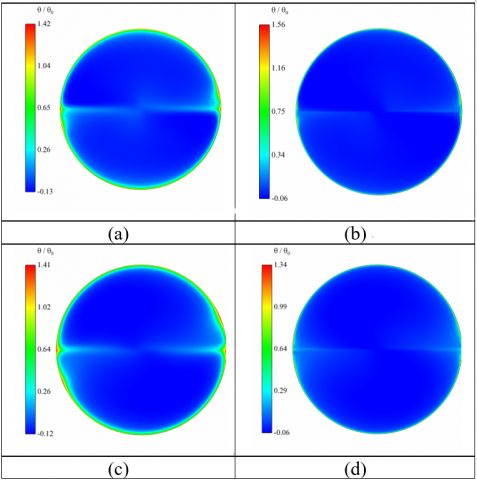Figure 12. Temperature contours for the fully developed flow at (a) Y = 4 and Re = 4000 (b) Y = 4 and Re = 30000 (c) Y = 10 and Re = 4000 (d) Y = 10 and Re = 30000

## 131.png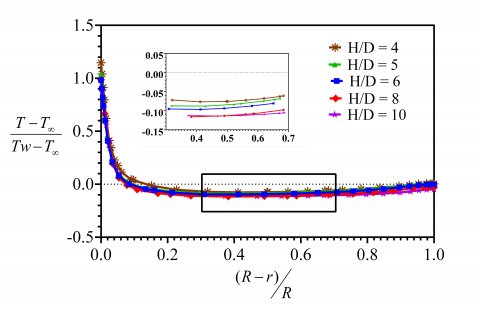(a)

## 132.png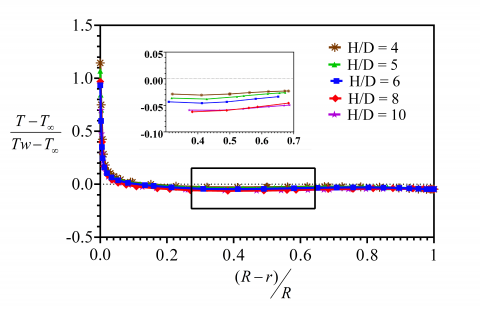(b)

Figure 13. Fluid temperature profile plotted at an angle of π/2 to the tape surface at (a) Re = 4000 (b) Re = 30000

The effect of twist ratio on the non dimensional temperature distribution in the fluid is shown in the Figure 13. The development of temperature gradients is observed at the low Reynolds number for twist ratio 4 as well as 10. The low inertial effects in the flow allow the formation of thermal boundary layer on the tape as well as on the tube surface. The thermal boundary reduces the heat transfer. The temperature rise is in the core area of the flow is a result of the rapid mixing of the fluid and the swirl. The tape fin effect produces the highest temperature in the tube tape contact area. The conduction in the tape increases the heat transfer rate.

5. Discussions

The introduction of twisted tape induces a strong swirl in the pipe flow. The swirl enhances the turbulence and allows superior mixing of the fluid inside the tube. The induced tangential velocity and radial velocity are foremost influencing parameters on the flow structure.  The tangential component reduces the maximum axial velocity and shifts the peak of the velocity towards the tube generating the non asymmetric velocity profile. The higher tangential velocity at the tube surface, sweeps the thermal boundary layer. This reduces the temperature gradients ultimately resulting in heat transfer rise. Therefore, the heat transfer enhancement which is a result of tangential velocity, increases with increasing Reynolds number and decreasing twist ratio.  The radial velocity being very small does not contribute significantly.  It causes the cross flow mixing and increases the rate of convection in the radial direction. Increase in average temperature of the fluid leads to the rise in the Nusselt number. Thus, the heat transfer mechanism and subsequent rise with the use of twisted tape depends on the components of the velocity in radial and tangential direction.

6. Conclusion

The heat transfer improvement in a pipe with the aid of twisted tape in turbulent flow regime is investigated numerically.  The study concludes that the secondary flow imparted by the twisted tape is in form of the radial and tangential velocity. The magnitude of tangential velocity increases with the distance from the center and its dominance in the flow field increases with Reynolds number. The vortex formation near the tape surface is observed for fully developed flow. Twisted tape alters the axial velocity profile by shifting the peak towards the tube with increasing Reynolds number at given twist ratio. This shift alters the structure thermal boundary layer. Thus, with rising Reynolds number, the heat transfer enhancement is also achieved. The Nusselt number and friction factor are correlated with the governing parameters and the results are in tune with the experimental results from the literature. The present work formulates and attempts the explanation of flow physic involved in the enhancement phenomenon of the heat transfer as well as of pressure drop with the use of twisted tape.  The aggregate effect of axial, tangential and radial convection and its impact on Nusselt number is offered here individually for each component of velocity. This helps us to explore the dominance of a particular component of velocity for the heat convection.

The present study includes the simulation using RANS turbulence model which is based on the time averaged quantities. The instantaneous data will give more elaborative picture of the flow characteristics. The LES (Large Eddy Simulation) technique is capable of producing instantaneous quantities which will help the deeper understanding of the complex flow behavior in case of the flow with twisted tape insert.

Nomenclature
 D Tube diameter (m) E Total Energy f Friction Factor H Twist pitch length (m) h Convective heat transfer coefficient (W/m2K) k Thermal conductivity of the material (W/m K) l Tube axial length (m) Nu Nusselt Number p Static Pressure(N/m2) Pr Prandtl Number q Heat Flux (W/m2) Re Reynolds Number T Temperature(K) u,v,w Components of velocity vector in Cartesian coordinates (m/s) Vm Mean velocity (m/s) Vr ,Vθ,Vz, Components of velocity vector in cylindrical coordinates (m/s) Y Twist Ratio Greek symbols µ Dynamic Viscosity (N.s/m2) ρ Density (kg/m3) θ Temperature difference across the local temperature and bulk temperature of the fluid (K) θ0 Temperature difference across the wall temperature and bulk temperature of the fluid (K) Subscripts i,j,k Tensor notations w Wall b Bulk Fluid Value
References

 Smithberg E., Landis F. (1964). Friction and forced convection heat-transfer characteristics in tubes with twisted tape swirl generators, Transactions ASME Journal of Heat Transfer, Vol. 86, pp. 39-48. DOI: 10.1016/0017-9310(84)90206-0

 Hong S.W., Bergles A.E. (1976). Augmentation of Laminar flow heat transfer in tubes by means of twisted Tape, Transactions ASME Journal of Heat Transfer, Vol.  98, pp. 251-256. DOI: 10.1016/0017-9310(84)90206-0

 Date A.W. (1974). Prediction of fully-developed flow in a tube containing a twisted-tape, International Journal of Heat and Mass Transfer, Vol. 17, pp. 845-859. DOI: 10.1016/0017-9310(74)90152-5

 Manglik R.M., Bergles A.E. (1993). Heat transfer and pressure drop correlations for twisted-tape inserts in isothermal tubes: Part I - Laminar flows, Transactions ASME Journal of Heat Transfer, Vol. 115, pp. 881-889. DOI: 10.1016/0017-9310(84)90206-0

 Manglik R.M., Bergles A.E. (1993). Heat transfer and pressure drop correlations for twisted-tape inserts in isothermal tubes: Part II: Transition and turbulent flows, Transactions ASME Journal of Heat Transfer, Vol. 115, pp. 890-896. DOI: 10.1016/0017-9310(84)90206-0

 Al-Fahed S., Chamra L.M., Chakroun W. (1998). Pressure drop and heat transfer comparison for both microfin tube and twisted-tape inserts in laminar flow, Experimental Thermal and Fluid Science, Vol. 18, pp. 323-333. DOI: 10.1016/S0894-1777(98)10037-7

 Bas H., Ozceyhan V. (2012). Heat transfer enhancement in a tube with twisted tape inserts placed separately from the tube wall, Experimental Thermal and Fluid Science, Vol. 41, pp. 51-58. DOI: 10.1016/j.expthermflusci.2012.03.008

 Wang L., Sundan B. (2002). Performance comparison of some tube inserts, International Communications in Heat and Mass Transfer, Vol. 29, pp. 45-56. DOI: 10.1016/S0735-1933(01)00323-2

 Klepper O.H. (1973). Heat Transfer performance of short twisted tapes, International Journal of Heat and Fluid Flow, Vol. 69, pp. 87-93. DOI: 10.1016/0142-727X(95)00096-9

 Saha, S.K. Dutta A., Dhal S.K. (2001). Friction and heat transfer characteristics of laminar swirl flow through a circular tube fitted with regularly spaced twisted-tape elements, International Journal of Heat and Mass Transfer, Vol. 44, pp. 4211-4223. DOI: 10.1016/S0017-9310(01)00077-1

 Saha S.K., Gaitonde U.N., Date A.W. (1990). Heat transfer and pressure drop characteristics of turbulent flow in a circular tube fitted with regularly spaced twisted-tape elements, Experimental Thermal and Fluid Science, Vol. 3, pp. 632-640. DOI: 10.1016/0894-1777(90)90080-Q

 Eiamsa-ard S., Promvonge P. (2005). Enhancement of heat transfer in a tube with regularly-spaced helical tape swirl generators, Solar Energy, Vol. 78, pp. 483-494. DOI: 10.1016/j.solener.2004.09.021

 Cazan R., Aidun C.K. (2009). Experimental investigation of the swirling flow and the helical vortices induced by a twisted tape inside a circular pipe, Physics of Fluids, Vol. 21. DOI: 10.1063/1.3085699

 Date A.W., Saha S.K. (1990). Numerical prediction of laminar flow, heat transfer characteristics in a tube fitted with regularly spaced twisted-tape elements, International Journal of Heat, Fluid Flow, Vol. 11, pp. 346-354. DOI: 10.1016/0142-727X(90)90058-J

 Chiu,Y.W., Jang J.Y. (2009). 3-D numerical and experimental analysis for thermal hydraulic characteristics of air flow inside a circular tube with different tube inserts, Applied Thermal Engineering, Vol. 29, pp. 250-258. DOI: 10.1016/j.applthermaleng.2008.02.030

 Rahimi M., Shabanian S.R., Alsairafi A.A. (2009). Experimental and CFD studies on heat transfer and friction factor characteristics of a tube equipped with modified twisted tape inserts, Chemical Engineering and Processing: Process Intensification, Vol. 48, pp. 762-770. DOI: 10.1016/j.cep.2008.09.007

 Wang Y., Hou M., Deng X., Li L., Huang C., Huang H., Huang,W. (2011). Configuration optimization of regularly spaced short-length twisted tape in a circular tube to enhance turbulent heat transfer using CFD modeling, Applied Thermal Engineering, Vol. 31, No. 6-7, pp. 1141-1149. DOI: 10.1016/j.applthermaleng.2010.12.009

 Ray S., Date A.W. (2001). Laminar flow and heat transfer through square duct with twisted tape insert, International Journal of Heat and Fluid Flow, Vol. 22, 460-472. DOI: 10.1016/S0142-727X(01)00078-9

 Lin Z.M., Wang L.B. (2009). Convective heat transfer enhancement in a circular tube using twisted tape, Transactions ASME Journal of Heat Transfer, Vol. 131. DOI: 10.1115/1.3122778

 Kitoh O. (1991). Experimental study of turbulent swirling flow in a straight pipe, Journal of Fluid Mechanics, Vol. 225, pp. 445-479. DOI: 10.1017/S0022112091002124

 Watanabe K.A., MoriY. (1983). Heat transfer augmentation in tubular flow by twisted tapes at high temperatures and optimum performance, Vol. 12, pp. 1-31.

 Sarma P.K., Subramanyam T., Kishore P.S., Rao V. D., Kakac S. (2002). A new method to predict convective heat transfer in a tube with twisted tape inserts for turbulent flow, International Journal of Thermal Sciences, Vol. 41, pp. 955-960. DOI: 10.1016/S1290-0729(02)01388-1

 Chattopadhyay H., Bandyopadhyay S., Bhattacharyya S. (2016). Numerical study on heat transfer enhancement through a circular duct fitted with centre-trimmed twisted tape, International Journal of Heat and Technology, Vol. 34, No. 3, pp. 401-406, September 2016. DOI: 10.18280/ijht.340308

 Kaliakatsos D., Cucumo M., Ferraro V., Mel M., Galloro A., Accorinti F. (2016). CFD Analysis of a Pipe Equipped with Twisted Tape, International Journal of Heat and Technology, Vol. 34, No. 2, pp. 172-180. DOI: 10.18280/ijht.340203

 Shukla A.K., Dewan A., (2016). Computational study on effects of rib height and thickness on heat transfer enhancement in a rib roughened square channel, Sādhanā - Academy Proc. in Engg. Sciences, Vol. 41, No. 6, pp. 667-678. DOI: 10.1007/s12046-016-0501-z